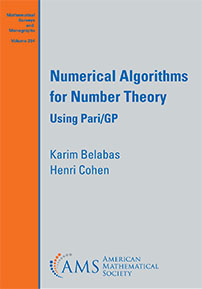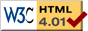This is the home page of the book Numerical Algorithms for Number Theory, written by Karim Belabas and Henri Cohen.

You can download all GP programs used in the book as a single file [TGZ | ZIP].This book presents multiprecision algorithms used in number theory and elsewhere, such as extrapolation, numerical integration, numerical summation (including Multiple Zeta Values and the Riemann-Siegel formula), evaluation and speed of convergence of continued fractions, Euler products and Euler sums, inverse Mellin transforms, and complex L-functions.

For each task, many algorithms are presented, such as Gaussian and doubly-exponential integration, Euler-MacLaurin, Abel-Plana, Lagrange, and Monien summation. Each algorithm is given in detail, together with a complete implementation in the free Pari/GP system. These implementations serve both to make even more precise the inner workings of the algorithms, and as a gentle introduction to advanced features of the Pari/GP language.

The book will be appreciated by anyone interested in number theory, specifically in practical implementations, computer experiments and numerical algorithms that can be scaled to produce thousands of digits of accuracy.

 Karim BelabasFri Feb 19 11:32:22 CET 2021